# Simulating Microfluidic Droplet Formation

Microfluidic droplet formation can be used for highly controlled nano- and micro- droplet manufacturing. These particles are formed by two immiscible phases dependent on low Reynolds number, equation 1. Droplet formations are formed using oil-in-water or water-in-oil for manipulating micro-litres, controlled mixing and encapsulation studies. The continuous phase is the medium in which droplets flow and the dispersed phase is the droplet fluid. In water-in-oil droplets, water is the dispersed phase and oil is the continuous phase.Equation 1: Reynolds equation where ρ is flow density, u is flow velocity, L is characteristic linear length (diameter of the channel) and μ is the flow dynamic viscosity1. Reynolds equation produces the dimensionless and unitless Reynolds number, Re. Reynolds number indicates whether flow is laminar or turbulent. Often laminar flow is defined as less than Re = 1000 where turbulent flow is greater2. Reynolds number limits, 1000, can differ depending on the CFD model such as open-flow, pipe or microfluidics. Flow is almost always laminar due to the small dimensional length in microfluidics.

Low Reynolds number, an indicator of laminar flow, is important to generate micro-droplets. The velocity ratio between the aqueous and oil phases contributes to the droplet size. Additionally, the microfluidic channel geometries manipulate the droplet size. In this demonstration, a capillary flow focusing device is implemented which forms droplets by focusing the flow of two immiscible fluids, figure 1.Figure 1: Example flow focusing device called capillary flow focusing from Wikipedia. The flow rate is controlled entering from the left-hand side for the dispersed fluid, H2O, and the flow rate is controlled equally for the top and bottom channels for the continuous phase, oil. The control over the flow rates is an important factor in droplet formation. Created by Kimbalm. Reused under Creative Commons Attribution-Share Alike 4.0 International.

The capillary flow focusing designed featured in figure 1 is one of many droplet formation devices present in the literature. An illustrated review of microfluidic droplet formation devices can be found by Zhu and Wang (2017)3. More flow focusing devices will be implemented in the near future.

## How Droplet Formation Is Simulated

The droplet formation is defined by the Cahn–Hilliard equation as shown in equation 2. The equation defines two phases of a binary fluid where in this simulation 0 is the continuous water phase and 1 is the dispersed oil phase.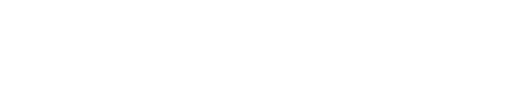Equation 2: Cahn-Hilliard equation where D∇2 is the diffusion coefficient with regards to the fluids and (c3 – c – γ ∇2 c) is the chemical potential where c is the chemical concentration4. The √γ gives the transition region lengths. This equation defines the phase separation.

Within BERNAISE, the Cahn-Hilliard equation is implemented as the sharp-interface model4, equation 2. This requires additional parameters between the two fluids such as the contact angle, surface tension, Interface Thickness and Phase Field Mobility Co-efficient.

Contact angle is the value at which the two fluids meet against a solid surface such as the microfluidic channel walls. This is important when simulating the pinching of dispersed fluid by the continuous phase. A low contact angle, < 120o, means droplets may not form5. Surface tension is important for the stability of the dispersed fluid, in this case the micro-droplet, within the continuous phase. A low surface tension could result in droplets collapsing into smaller poly-dispersed solutions. If the physical interface thickness is resolved by the phase field (for example, the droplets are too small to exist), a diffuse model might be more appropriate. As the simulation is aiming to solve a microfluidic droplet with defined edges, a sharp-interface model is appropriate.

•Phase Field Mobility Coefficient. The Mobility co-efficient is the diffusion coefficient of the two fluids important for droplet movement and stability. The yellow arrows represent the diffusion of the dispersed phase in the continuous phase, blue is the dispersed phase and red is the continuous phase.
•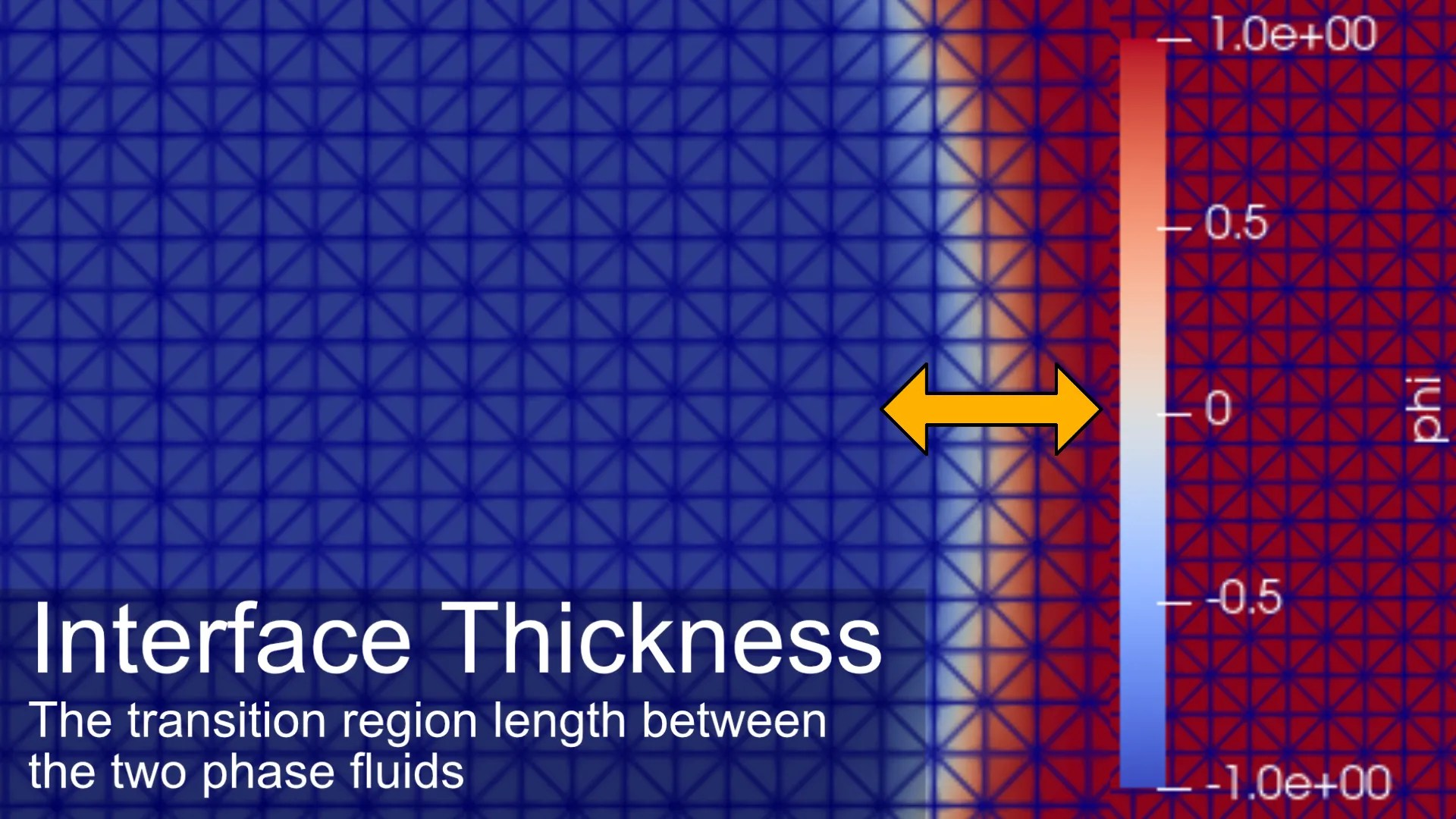Phase Field Interface Thickness. The interface thickness acts as the transition region between the phase fields. The transition region is dependent cell size where the transition length must exceed the mesh cell size to be calculated. The yellow arrow represents the transition region length, white is the computed transition, blue is the dispersed phase and red is the continuous phase.

Phase field requires two additional parameters, Interface Thickness and Phase Field Mobility Co-efficient. The Interface Thickness parameter acting as the transition region length between the two phases. This value is limited by the mesh cell size, a limiting factor of the simulation. The diffusion coefficient of the fluids is defined using the Phase Field Mobility Co-efficient. It is fundamental the Interface Thickness and Phase Field Mobility Co-efficient are carefully set. If the values are too small, the simulation will fail as it cannot compute the values. If the values are too large, the droplets will be unstable and break up in the simulation. The Interface Thickness and Phase Field Mobility Co-efficient are defined in table 2. A detailed explanation of both parameters is described in Asger Bolet’s, Gaute Linga’s and Joachim Mathiesen’s paper as well as Comsol Two-Phase Flow Modelling Guidelines.Equation 3: Navier-Stokes equation describing the force balance at a given point within a fluid4,6. The du/dt is the time dependent velocity, u is the velocity term, v is the dynamic viscosity, p is fluid density with P being pressure.

The movement of the phase-field fluids is determined using the Navier-Stokes equations, equation 3. In particular, the Incompressible Navier–Stokes equations where flow is governed by the fluid viscosity, density, velocity, pressure and internal stress4,6. Here, additional trial and test terms for the phase field are added to the PDE solver retaining the chemical potential and diffusivity as well as the phase field properties such as contact angle.

## Problem Specific Variables

The values presented were adapted from a YouTube tutorial video by AGM ONLINE SIMULATION along with a similar mesh. The values can be easily adapted to other droplet formation problems. The values act as a proof of principle for replicating similar results and dynamics seen in the reference tutorial video.

Additional parameters specific to the phase field need to be assigned…

## Software

BERNAISE, Binary ElectRohydrodyNAmIc SolvEr, is an open-source, free CFD solver created in FEniCS – a solver for partial differential equations (PDEs). BERNAISE is a flexible high-level solver of electrohydrodynamic flows in complex geometries. It is written in Python and built on the FEniCS project, which in turn effectively interfaces to optimized linear algebra backends such as PETSc. The solver is described in Asger Bolet’s, Gaute Linga’s and Joachim Mathiesen’s paper.

This particular version of BERNAISE has been modified by myself to use .XDMF computer aided design (CAD) meshes as well as has been focused towards microfluidic examples. Due to the large size of the simulation, additional computational power may be required. A tutorial is available on the BERNAISE GitHub.

## Step By Step

1. Convert the CAD into a mesh file for FEniCS and BERNAISE to read and carry out simulations. A full description of the steps is available for 2D and 3D CAD conversion on the BERNAISE wiki. Briefly, produce the mesh in an appropriate CAD program and export as a .STEP file. Load the Use meshio and convert the .gmsh file to .XDMF files for the mesh and boundary domain labels.
2. Assign parameters in the problem python file. In this case, the problem has been called flow_focusing and the problem specific parameters are defined in the problem() function. More example problems can be found in the problems folder. In particular, the boundary labels mentioned in step 1 for assigning boundary properties as well as the Problem Specific Variables mentioned earlier in this blog. It is important to define the continuous and dispersed phase.
3. Run the problem from the command prompt `python sauce.py problem=flow_focusing`
4. View the exported .XDMF results in ParaView.

## Reviewing The Results

BERNAISE has presented a platform that can model droplet formation at the micro-scale. The formation of the droplets has shown to be similar to that of the reference YouTube video. Exact similarities are not expected between the two models due to differences in the mesh used and differences in the phase field calculations. The simulation is an approximation of the expected real-world result based on the parameters used. The result could be improved by adjusting the mesh density at the expense of computational requirements as well as adjusting phase field parameters such as Phase Field Mobility Co-efficient and Interface Thickness.

•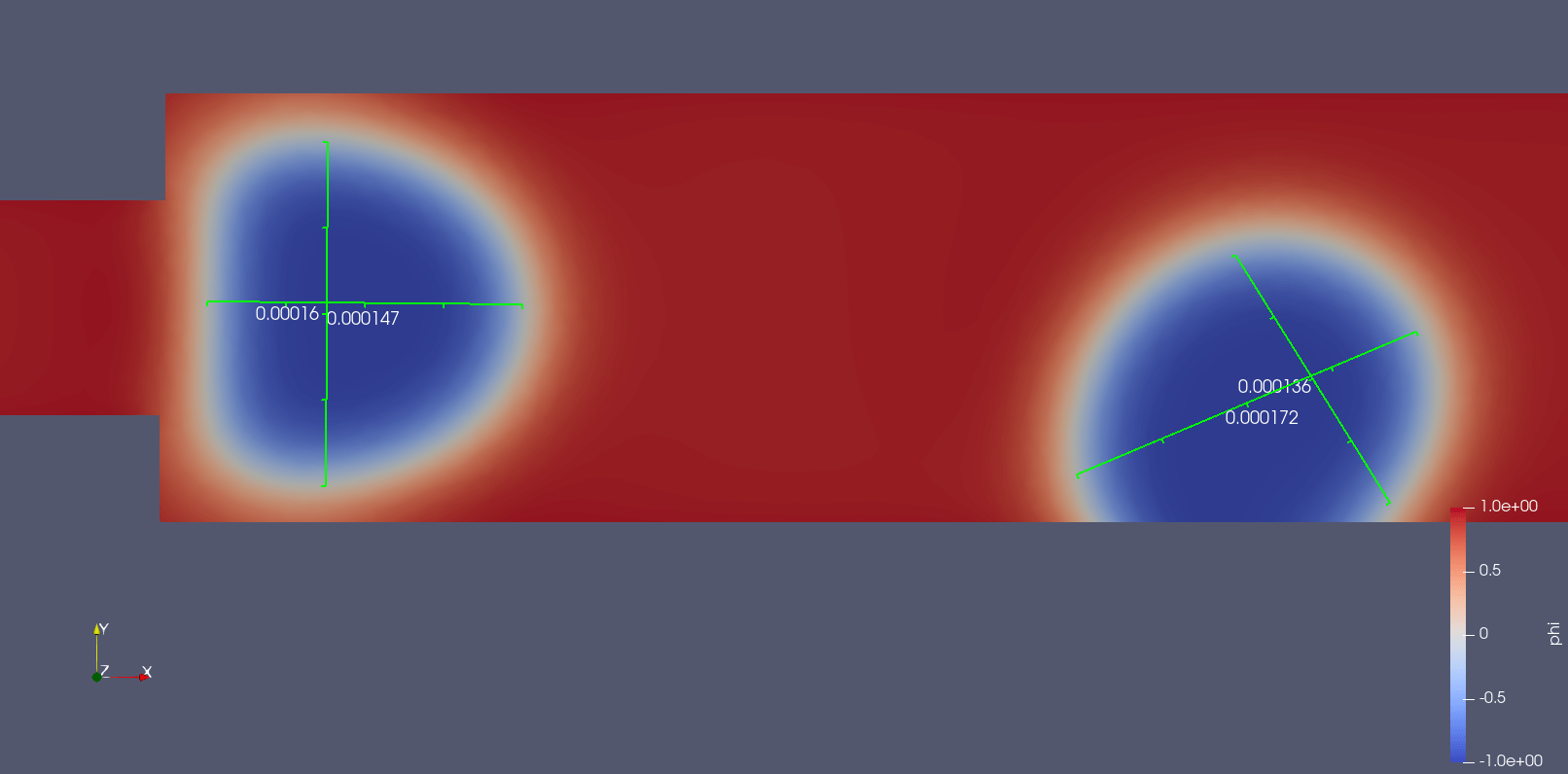Phase field of water-in-oil droplets. The droplets are around 150 µm. Units displayed are in metres. Blue is -1 and red is 1 indicating the two immiscible fluids. The white halo is the transition zone.
•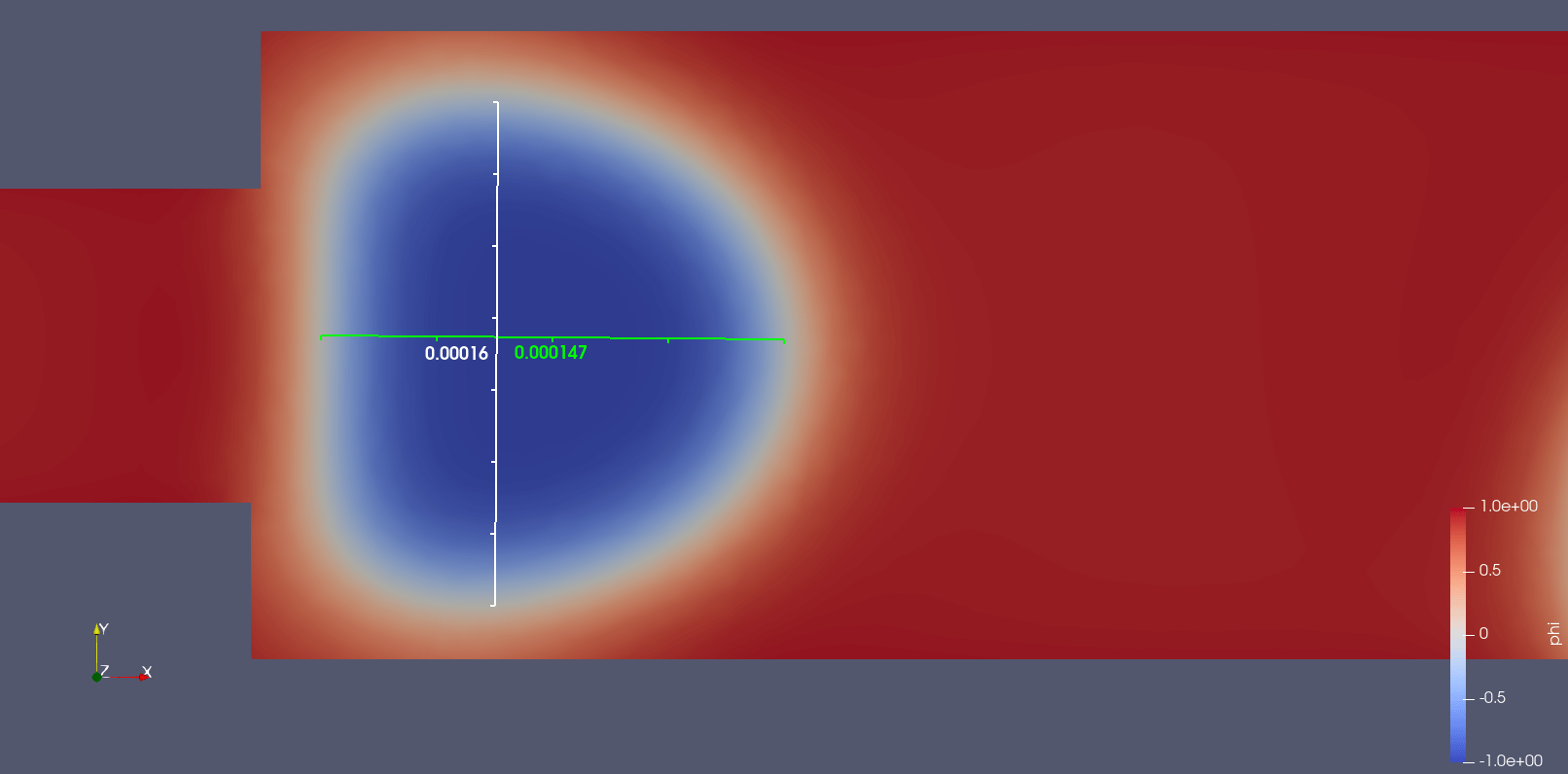Phase field of water-in-oil droplets. The droplets are around 150 µm. Units displayed are in metres. Blue is -1 and red is 1 indicating the two immiscible fluids. The white halo is the transition zone.
•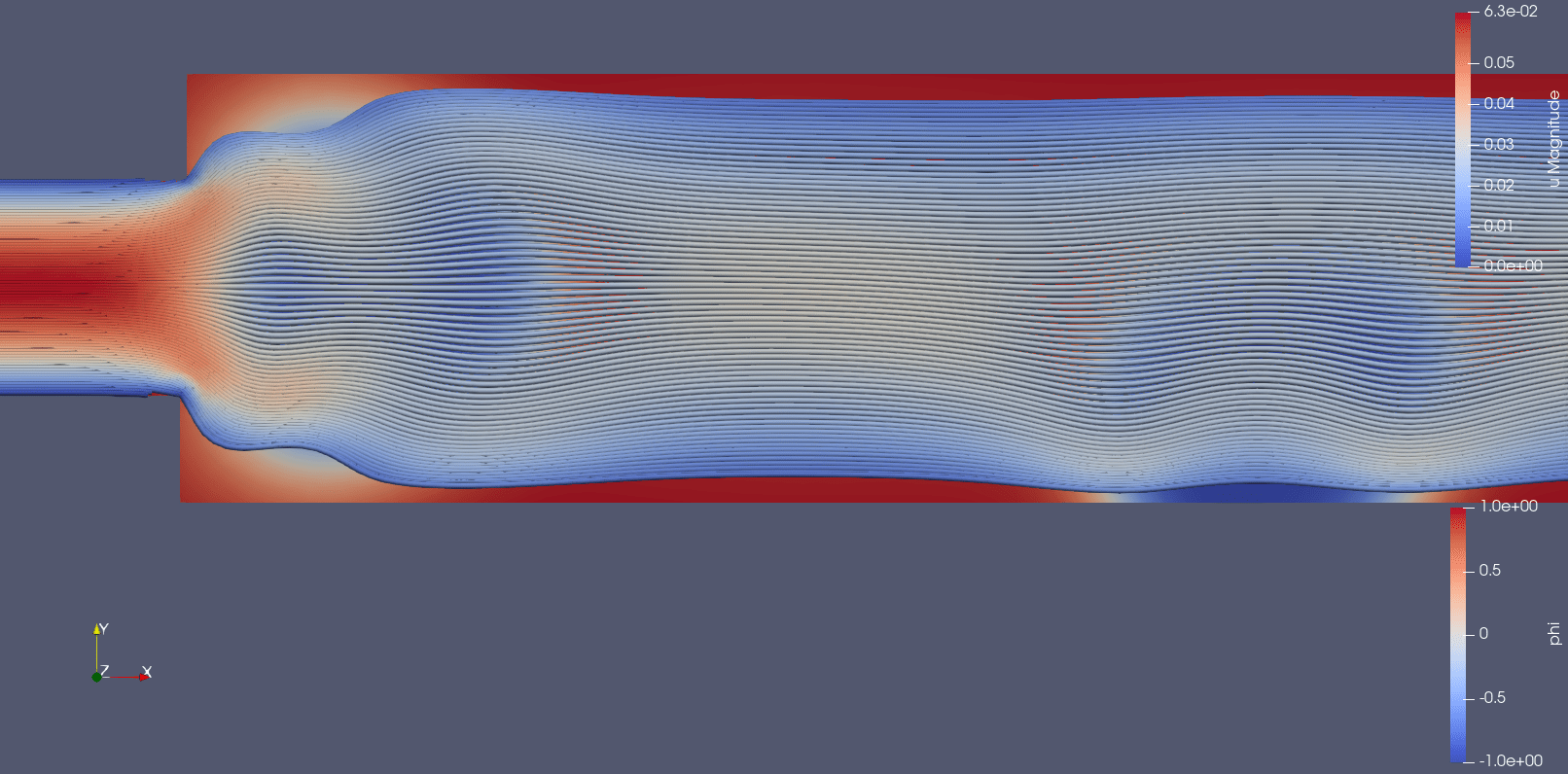Streamlines overlaid on phase field of water-in-oil droplets. Blue is -1 and red is 1 indicating the two immiscible fluids. The white halo is the transition zone. The stream lines are red to blue from 0.063 m / s to 0 m / s
•Streamlines at the inlet of the capillary flow focusing device. The stream lines are red to blue from 0.063 m / s to 0 m / s.
•Streamlines overlaid on phase field of water-in-oil droplets at the inlet of the capillary flow focusing device. Blue is -1 and red is 1 indicating the two immiscible fluids. The white halo is the transition zone. The stream lines are red to blue from 0.063 m / s to 0 m / s.

The formed droplet had the expected size of around 150 μm which was determined by the geometry of the channel. Further studies could optimise the droplet size by changing the geometries or flow ratios between the continuous and dispersed phases. Additionally, continuous and dispersed phase properties could be adjusted such as viscosity, surface tension and contact angle. Adjustments to the continuous and dispersed phase properties would mean real-world adjustments to the experiment. The adjustments to the experiment could be adjusting the surface properties of the microfluidic device or changing the exact continuous and dispersed phase fluids. The changes in the real-world experiment may not be possible due to bio-compatibility or chemical stability.

## Conclusion

Presented is a guide for simulating microfluidic droplet formation using a capillary flow focusing device with demonstration data. The droplet simulation makes assumptions using the sharp-interface model which is appropriate for simulating real-world microfluidic droplet formation. It should be noted that simulations will always be limited by the parameters used and may not be accurate to real-world problems. However, parameter sweeps can test many parameters in an efficient and quick method compared to experiments In Vitro. The efficiency saves experimental time, supply costs such as microfluidic manufacturing and experimental supply costs. Ultimately, it is an important part of the “tool kit” for developing any microfluidic platform by supporting the In Vitro experiments.

## References

1. Fluigent (2021) Basic Properties of Microfluidic Flows. [Accessed on 25th August 2021] Physics of Microfluidics – Basic Properties of Microfluidic Flows | Fluigent
2. C.T. Shin U. Ghia, K.N. Ghia. High-Resolution for incompressible flow using the Navier-Stokes equations and the multigrid method. J. Comput. Phys., 48:387–411, 1982.
3. Zhu, P., & Wang, L. (2017). Passive and active droplet generation with microfluidics: a review. Lab on a Chip, 17(1), 34–75. https://doi.org/10.1039/C6LC01018K
4. Linga, G., Bolet, A., & Mathiesen, J. (2019). Bernaise: A flexible framework for simulating two-phase electrohydrodynamic flows in complex domains. Frontiers in Physics, 7(MAR). https://doi.org/10.3389/fphy.2019.00021
5. Eggersdorfer, M. L., Seybold, H., Ofner, A., Weitz, D. A., & Studart, A. R. (2018). Wetting controls of droplet formation in step emulsification. Proceedings of the National Academy of Sciences of the United States of America, 115(38), 9479–9484. https://doi.org/10.1073/pnas.1803644115
6. Ladyzhenskaya, O. A. (1975). Mathematical analysis of navier-stokes equations for incompressible liquids. Annual Review Fluid Mechanics, v, 249–272. https://doi.org/10.1146/annurev.fl.07.010175.001341